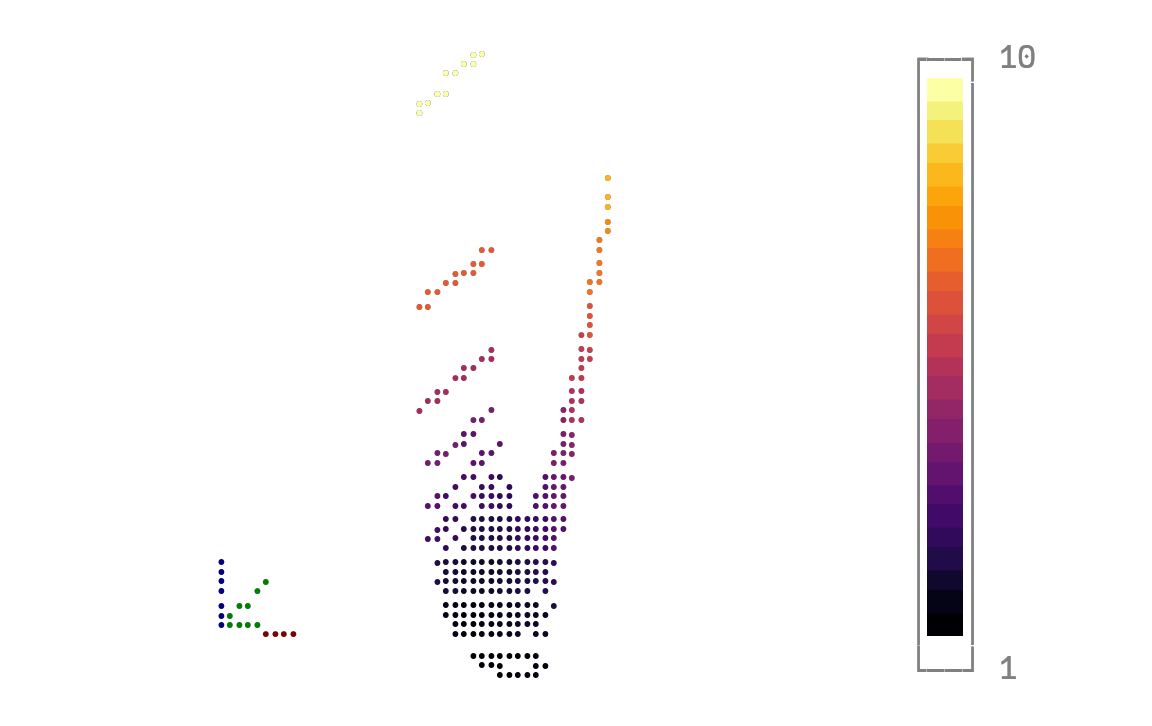# 3D surface with axis guidesusing Plots
unicodeplots()
func(x, a) = begin
1 / x + a * x ^ 2
end
xs = collect(0.1:0.05:2.0)
as = collect(0.2:0.1:2.0)
x_grid = [x for x = xs for y = as]
a_grid = [y for x = xs for y = as]
plot(x_grid, a_grid, func.(x_grid, a_grid), st = :surface, xlabel = "longer xlabel", ylabel = "longer ylabel", zlabel = "longer zlabel")This page was generated using DemoCards.jl and Literate.jl.